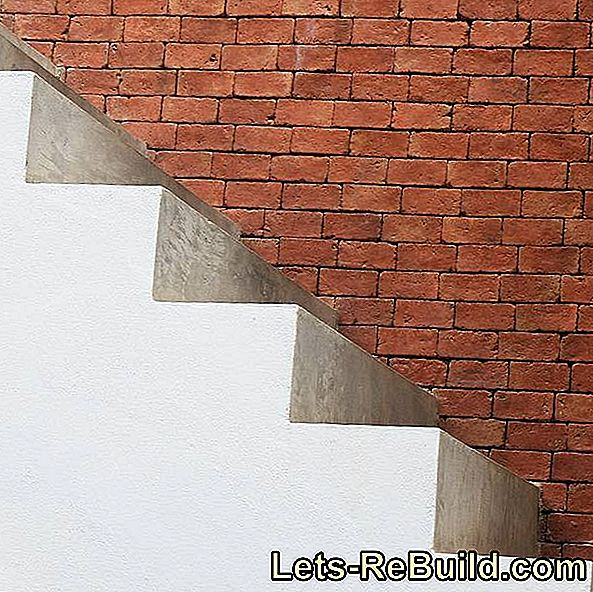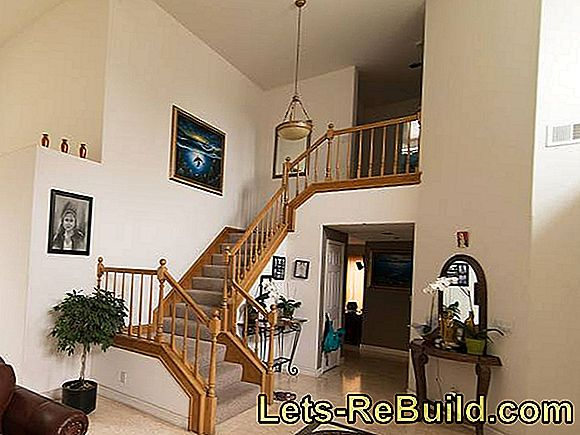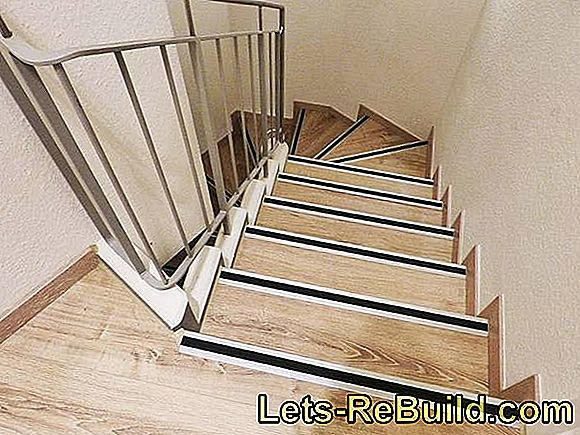Calculate stairs

Calculate stairs - instructionsYou have to calculate and plan a staircase? That is possible. However, there is more to be considered than perhaps thought at the beginning of such a project.

After all, the DIN 18065 regulates quite specifically, which scope you have legally in terms of dimensions. This applies to residential buildings with up to two apartments and inside of apartments, so almost all private staircase projects.

If you want to calculate a staircase, you are probably concerned with the number, height and depth of the steps, perhaps also the slope and the running width. There are a number of formulas and rules that you will find in this guide.

Also online, there are some computers with which you can calculate a staircase. However, the most important technical terms around the stairs should be known and some basic dimensions. You can find out all this here.

Contents: Calculate stairs

• The main concepts of a staircase are briefly explained
• Stairs DIN 18065 - regulations for attention
• Stairs calculate formulas and rules
• Calculate stairs - Example of the formula application and tips

The main concepts of a staircase are briefly explained• commencement = first step (below); first climb
• performances = Step depth, however, only up to the point where the next step starts above it (in case of overhang)
• exit = Entrance area at the top of the stairs
• Usable running width = Step width minus width of handrails incl. Brackets, ie the width, which is also freely usable in the height.
• incremental = Length of one step, measured from the heel edge to the heel edge (general average is 63 cm for an average adult)
• pitch = in this context, the clear height of a step
• riser = vertical back wall between two steps in a closed staircase, d. H. there are no risers in an open staircase
• tread = all stages between entry and exit
• ¼ spiral staircase = this stair once turns 90 degrees from the entrance to the exit
• ½ spiral staircase = these stairs turns 180 degrees from step to step

Stairs DIN 18065 - regulations for attention

The DIN 18065 differentiates between necessary under construction law stairs and additional, so legally not necessary stairs. These include stairs within an apartment and floor, ladder and other steep stairs that tap into, for example, an attic.

For the stairs required by construction law (escape routes) a useable running width of at least 80 cm is prescribed. The gradients (clear height between the treads) must be at least 14 and a maximum of 20 cm high, the appearance (step depth without projection) must / must be at least 23 and a maximum of 37 cm.

For stairs that are not necessary under construction law, the usable running width is at least 50 cm, the pitch at least 14 but not more than 21 cm, and the entrance at least 21 but not more than 37 cm.

Stairs calculate formulas and rules

• Number of performances (levels) = height to be overcome (eg floor height): slope height
• Appearance width (depth of steps) = Increment - 2 x grade height
• Run length of the stairs = Entrance width (depth of steps) x number of appearances (steps)
• Slope of the stairs = height to be overcome: run length
• slope height = height to be overcome: number of performances (levels)
• Schrittmaßregel: 2 x incline (clear step height) + 1 x step (step depth) = increment
• Convenience rule: Tread width (depth of steps) - pitch height = 12 cm
• security Regel: Incline height + width of step (depth of steps) = 46 cm
• pass line = A path is assumed exactly in the middle of the steps and if necessary the podium. On the running line, even with coiled stairs, the DIN regulations must be adhered to.

Calculate stairs - Example of the formula application and tips

Good to know: A total incline of 30% and steps of 27 cm depth and 18 cm height are considered very comfortable. Therefore, these values, in staircase planning as an approach to applying one or the other staircase formula, are definitely recommended.

One of the dimensions of the stairs known from the beginning is the height to be overcome, sometimes even the only one fixed. Starting from this value, the number of steps, that is to say the appearances and the pitch, shall be calculated.With enough space podiums can be incorporated. This makes all levels the same, which facilitates the comfortable ascent.

Example for stair calculation

You have to overcome a height of 250 cm in the attic and now want to know how many stages (performances) you need to know.

You apply the following formula:

Number of appearances (levels) = height to be overcome: Incline height

250 cm: 18 cm (known as comfortable) = 13,89 (14 steps)

This means that you plan 14 appearances (levels), as it is generally rounded up or down if no smooth number comes out of it. Unless you want to calculate a garden staircase and can easily create a small leveling in the terrain, d. H. slightly change the height to be overcome.

Since you need all the steps at the same distance, so that no pitfalls caused by different heights, you want to know in the next step, then how the actual pitch height fails.

For this you use this formula:

Incline height = height to be overcome: number of occurrences (steps)

250 cm: 14 gigs = 17,86 cm incline height

Next, you want to calculate the run length of the stairs to see if it also fits the space available, for example, to calculate or plan a staircase.

In that case, this formula is used:

Run length of stairs = width of step (depth of steps) x number of steps (steps)

27 cm (we know it as comfortable) x 14 gigs = 378 cm run lengthA ½ spiral staircase saves space, but has different steps and is not comfortable to walk.

If the staircase does not fit in this form, with really small differences there is the possibility to give up on comfort and to slightly change the dimensions (in the context of DIN 18065) ​​until it fits.

If the deviations are more significant, you could calculate a different shaped staircase - with or without a pedestal, but in any case a spiral staircase, which then becomes shorter but wider.

These staircases have the same formulas, but in this case you should make a drawing to calculate the stairs - a simple sketch is enough. Because to the determined values, parts to be added, such as possibly a stairwell or the pedestal come into play. All this is entered in the drawing and can be divided into sections for calculation and control, calculated and added accordingly.

More tips:

Think of enough space, eg. B. without opening doors directly before the start and after the exit.

The following applies to the opening of the ceiling: The headroom corresponds to the DIN, if it is equal to> = 200 cm high.

Similar sites

• Wooden stairs and solid wood stairs
• Calculate concrete fall
• Rediscover the roof
• Build stable and simple wooden staircase yourself
• Stair renovation for DIY - stair kit and stair renovation